A body takes time t to reach the bottom of an inclined plane of angle θ with the horizontal. If the plane is made rough, time taken now is 2t. The coefficient of friction of the rough surface is

(1) $\frac{3}{4}\mathrm{tan}\theta$

(2) $\frac{2}{3}\mathrm{tan}\theta$

(3) $\frac{1}{4}\mathrm{tan}\theta$

(4) $\frac{1}{2}\mathrm{tan}\theta$

Concept Questions :-

Friction
High Yielding Test Series + Question Bank - NEET 2020

Difficulty Level:

A block is kept on an inclined plane of inclination θ of length l. The velocity of particle at the bottom of inclined is (the coefficient of friction is μ)

(1) $\sqrt{2gl\left(\mu \mathrm{cos}\theta -\mathrm{sin}\theta \right)}$

(2) $\sqrt{2gl\left(\mathrm{sin}\theta -\mu \mathrm{cos}\theta \right)}$

(3) $\sqrt{2gl\left(\mathrm{sin}\theta +\mu \mathrm{cos}\theta \right)}$

(4) $\sqrt{2gl\left(\mathrm{cos}\theta +\mu \mathrm{sin}\theta \right)}$

Concept Questions :-

Friction
High Yielding Test Series + Question Bank - NEET 2020

Difficulty Level:

A block of mass m lying on a rough horizontal plane is acted upon by a horizontal force P and another force Q inclined at an angle θ to the vertical. The block will remain in equilibrium, if the coefficient of friction between it and the surface is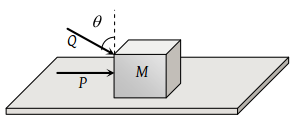(1) $\frac{\left(P+Q\mathrm{sin}\theta \right)}{\left(mg+Q\mathrm{cos}\theta \right)}$

(2) $\frac{\left(P\mathrm{cos}\theta +Q\right)}{\left(mg-Q\mathrm{sin}\theta \right)}$

(3) $\frac{\left(P+Q\mathrm{cos}\theta \right)}{\left(mg+Q\mathrm{sin}\theta \right)}$

(4) $\frac{\left(P\mathrm{sin}\theta -Q\right)}{\left(mg-Q\mathrm{cos}\theta \right)}$

Concept Questions :-

Friction
High Yielding Test Series + Question Bank - NEET 2020

Difficulty Level:

A block of mass 0.1 kg is held against a wall by applying a horizontal force of 5 N on the block. If the coefficient of friction between the block and the wall is 0.5, the magnitude of the frictional force acting on the block is

(1) 2.5 N

(2) 0.98 N

(3) 4.9 N

(4) 0.49 N

Concept Questions :-

Friction
High Yielding Test Series + Question Bank - NEET 2020

Difficulty Level:

What is the maximum value of the force F such that the block shown in the arrangement, does not move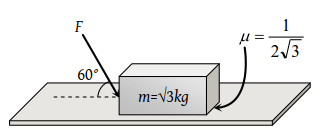(1) 20 N

(2) 10 N

(3) 12 N

(4) 15 N

Concept Questions :-

Friction
High Yielding Test Series + Question Bank - NEET 2020

Difficulty Level:

A body of mass m rests on horizontal surface. The coefficient of friction between the body and the surface is μ. If the mass is pulled by a force P as shown in the figure, the limiting friction between body and surface will be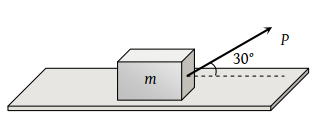(1) μmg

(2) $\mu \text{\hspace{0.17em}}\left[mg+\left(\frac{P}{2}\right)\right]$

(3) $\mu \text{\hspace{0.17em}}\left[mg-\left(\frac{P}{2}\right)\right]$

(4) $\mu \text{\hspace{0.17em}}\left[mg-\left(\frac{\sqrt{3}\text{\hspace{0.17em}}P}{2}\right)\right]$

Concept Questions :-

Friction
High Yielding Test Series + Question Bank - NEET 2020

Difficulty Level:

A 40 kg slab rests on a frictionless floor as shown in the figure. A 10 kg block rests on the top of the slab. The static coefficient of friction between the block and slab is 0.60 while the kinetic friction is 0.40. The 10 kg block is acted upon by a horizontal force 100 N. If g = 9.8 m/s2, the resulting acceleration of the slab will be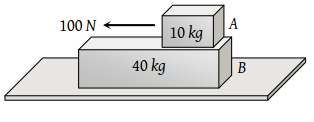(1) 0.98 m/s2

(2) 1.47 m/s2

(3) 1.52 m/s2

(4) 6.1 m/s2

High Yielding Test Series + Question Bank - NEET 2020

Difficulty Level:

A body of weight 50 N placed on a horizontal surface is just moved by a force of 28.2 N. The frictional force and the normal reaction are(1) 10 N, 15 N

(2) 20 N, 30 N

(3) 2 N, 3 N

(4) 5 N, 6 N

High Yielding Test Series + Question Bank - NEET 2020

Difficulty Level:

Block A weighing 100 kg rests on a block B and is tied with a horizontal string to the wall at C. Block B weighs 200 kg. The coefficient of friction between A and B is 0.25 and between B and the surface is 1/3. The horizontal force P necessary to move the block B should be (g = 10 m/s2)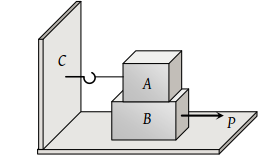(1) 1150 N

(2) 1250 N

(3) 1300 N

(4) 1420 N

High Yielding Test Series + Question Bank - NEET 2020

Difficulty Level:

A rough vertical board has an acceleration ‘a’ so that a 2 kg block pressing against it does not fall. The coefficient of friction between the block and the board should be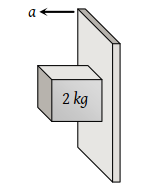(1) > g/a

(2) < g/a

(3) = g/a

(4) > a/g